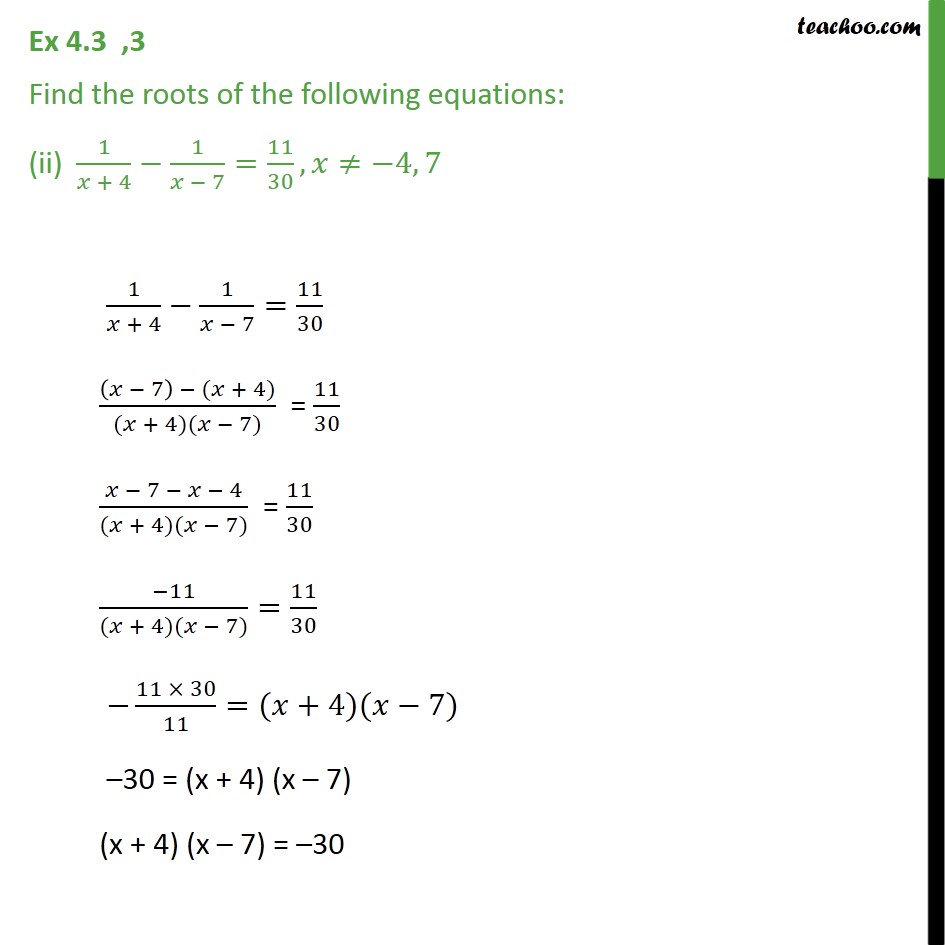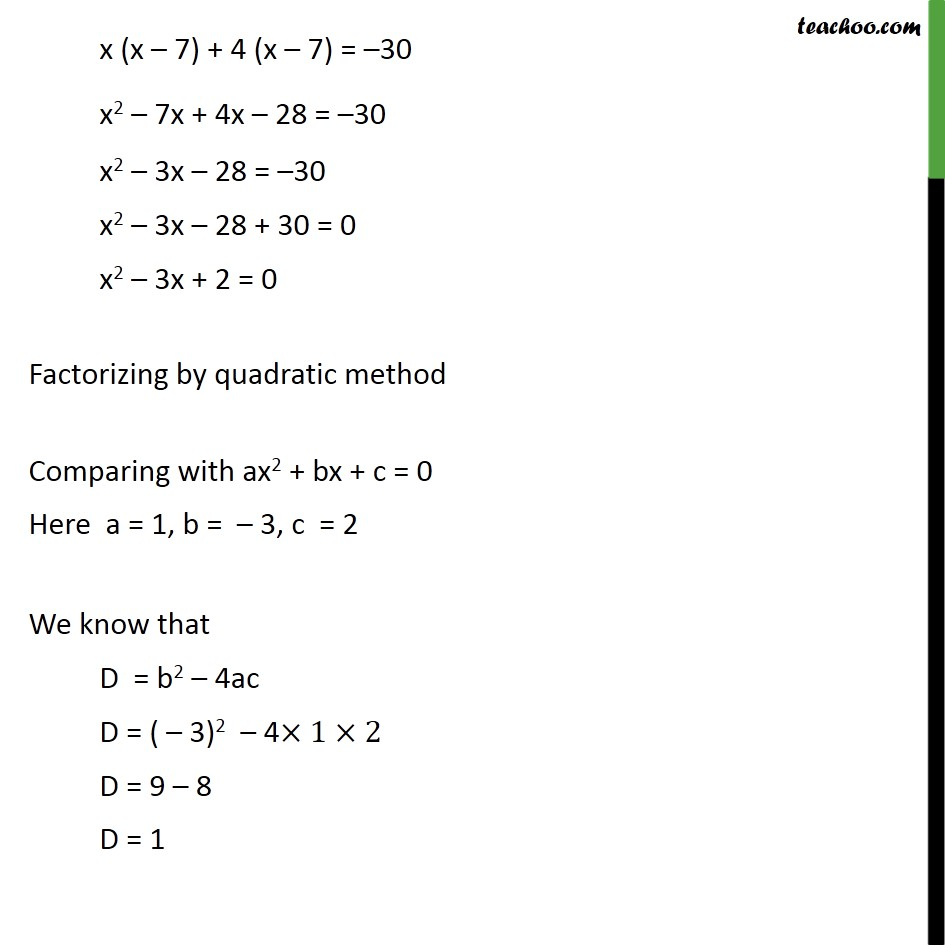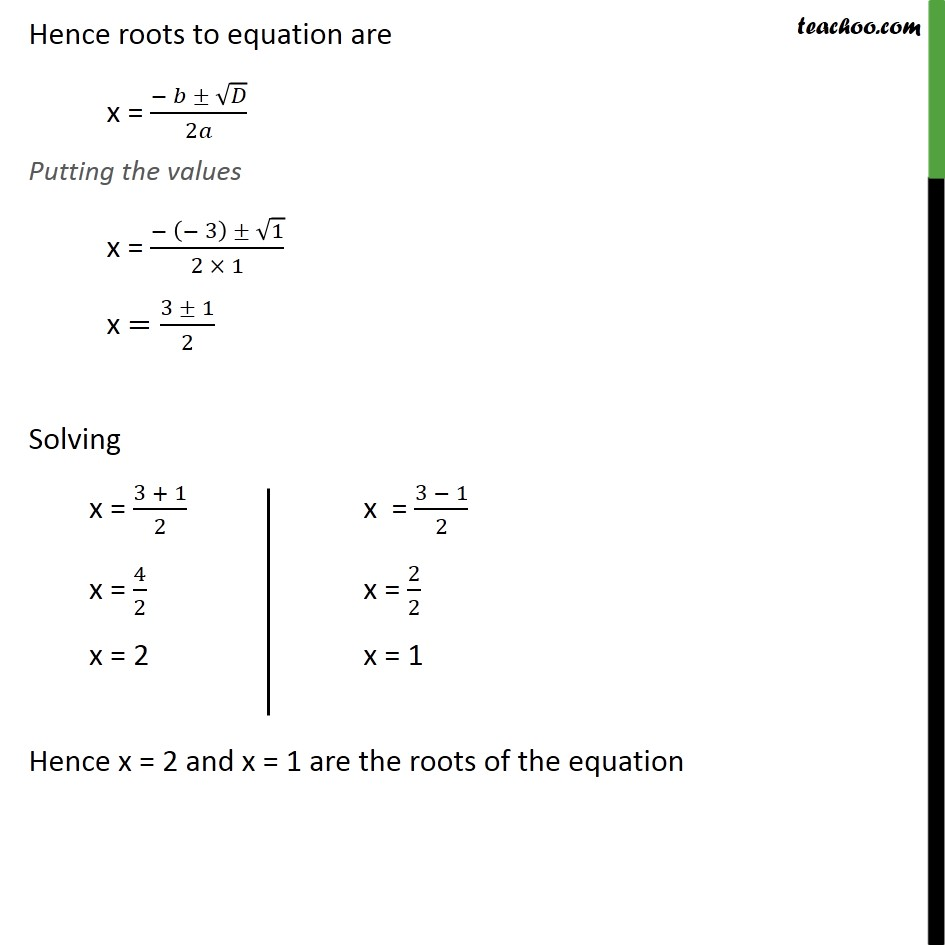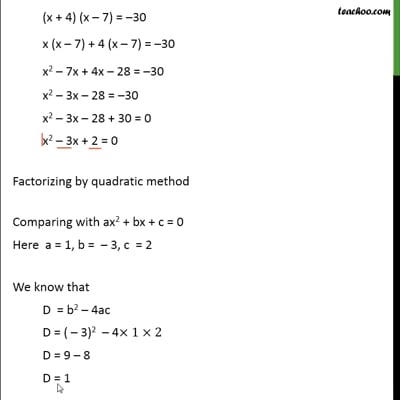Ex 4.3

Chapter 4 Class 10 Quadratic Equations
Serial order wiseThis video is only available for Teachoo black users

Introducing your new favourite teacher - Teachoo Black, at only ₹83 per month

### Transcript

Ex 4.3 ,3 Find the roots of the following equations: (ii) 1/(𝑥 + 4)−1/(𝑥 − 7)=11/30,𝑥≠−4, 7 1/(𝑥 + 4)−1/(𝑥 − 7)=11/30 ((𝑥 − 7) − (𝑥 + 4))/((𝑥 + 4)(𝑥 − 7)) = 11/30 (𝑥 − 7 − 𝑥 − 4)/((𝑥 + 4)(𝑥 − 7)) = 11/30 (−11)/((𝑥 + 4)(𝑥 − 7))=11/30 −(11 × 30)/11=(𝑥+4)(𝑥−7) –30 = (x + 4) (x – 7) (x + 4) (x – 7) = –30 x (x – 7) + 4 (x – 7) = –30 x2 – 7x + 4x – 28 = –30 x2 – 3x – 28 = –30 x2 – 3x – 28 + 30 = 0 x2 – 3x + 2 = 0 Factorizing by quadratic method Comparing with ax2 + bx + c = 0 Here a = 1, b = – 3, c = 2 We know that D = b2 – 4ac D = ( – 3)2 – 4×1×2 D = 9 – 8 D = 1 Hence roots to equation are x = (− 𝑏 ± √𝐷)/2𝑎 Putting the values x = (− (− 3) ± √1)/(2 × 1) x =(3 ± 1)/2 Solving Hence x = 2 and x = 1 are the roots of the equation